# Triangles and Its Properties Class 7 Notes: Chapter 6

## Introduction

### Triangle

• A triangle is a closed curve made of three line segments.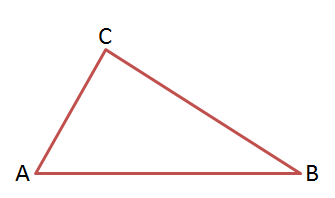• It has three:Sides:
(i) Sides: ¯¯¯¯¯¯¯¯AB, ¯¯¯¯¯¯¯¯BC and ¯¯¯¯¯¯¯¯CA
(ii) Angles: ∠BAC, ∠ACB and ∠CBA
(iii) Vertices: A, B and CTo know more about Triangle, visit here.

## Important Lines in a Triangle

### Median

• Median is the line that connects a vertex of a triangle to the mid-point of the opposite side.
• In the given figure, ¯¯¯¯¯¯¯¯¯AD is the median, joining the vertex A to the midpoint of ¯¯¯¯¯¯¯¯BC.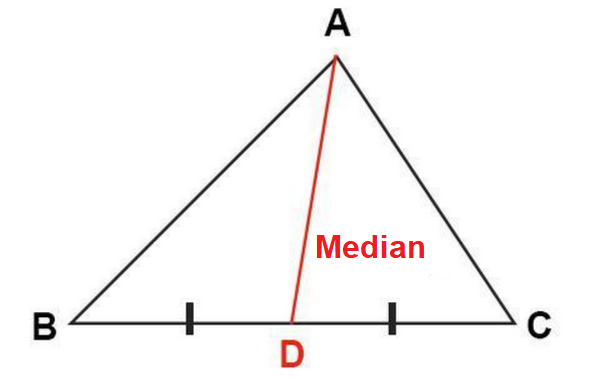### Altitude

• An altitude is a line segment through a vertex of the triangle and perpendicular to a line containing the opposite side.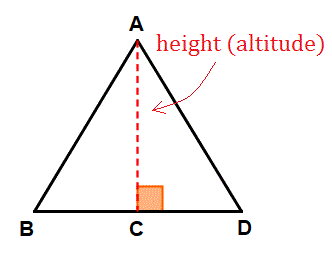## Sides Also Have Constraints

### Sum of the lengths of two sides of a triangle

• The sum of the lengths of any two sides of a triangle is greater than the third side.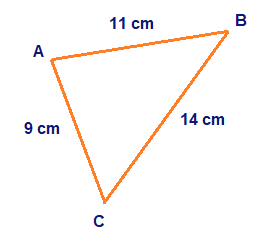In the above triangle,
9+11=20 >14
11+14=25 >9
9+14=23 >11

### Difference between lengths of two sides of a triangle

• The difference between lengths of any two sides is smaller than the length of the third side.• In the above triangle,
11 – 9 = 2 < 14
14 – 11 = 3 < 9
14 – 9 = 5 < 11
• Using the concept of sum of two sides and difference of two sides, it is possible to determine the range of lengths that the third side can take.

## Triangle Properties

### Angle sum property of a triangle

• The total measure of the three angles of a triangle is 1800.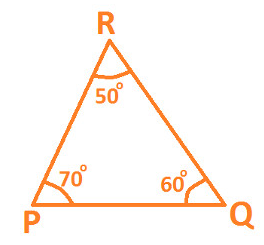• In △PQR,
∠RPQ+∠PQR+∠QRP
=70+ 60+ 50= 1800

#### For More Information On Angle Sum Property Of A Triangle, Watch The Below Video.To know more about Properties of Triangle, visit here.

### Exterior angle of a triangle and its property

• An exterior angle of a triangle is equal to the sum of its interior opposite angles.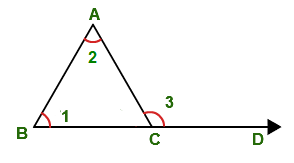In the given figure, ∠1+∠2=∠3.

#### For More Information On Exterior Angle Theorem, Watch The Below Video.### Pythagoras Theorem

• The side opposite to the right angle in a right-angled triangle is called the hypotenuse.
• The other two sides are known as legs of the right-angled triangle.
• In a right-angled triangle, square of hypotenuse is equal to the sum of squares of legs.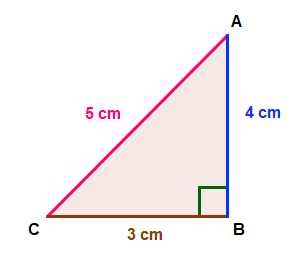AC2=AB2+BC2
⇒52=42+32
• If a triangle holds Pythagoras property, then it is a right-angled triangle.To know more about Pythagoras Theorem, visit here.

### Properties of isosceles and equilateral triangles

Properties of Isosceles Triangle

• Two sides are equal in length.
• Base angles opposite to the equal sides are equal.

To know more about Properties of Isosceles Triangle, visit here.
Properties of Equilateral Triangle

• All three sides are equal in length.
• Each angle equals to 600.

To know more about Properties of Equilateral Triangle, visit here.

## Classification of Triangles

### Classification of triangles based on sides

• Equilateral triangle: A triangle in which all the three sides are of equal lengths.
• Isosceles triangle: A triangle in which two sides are of equal lengths.
• Scalene Triangle: A triangle in which all three sides are of different length.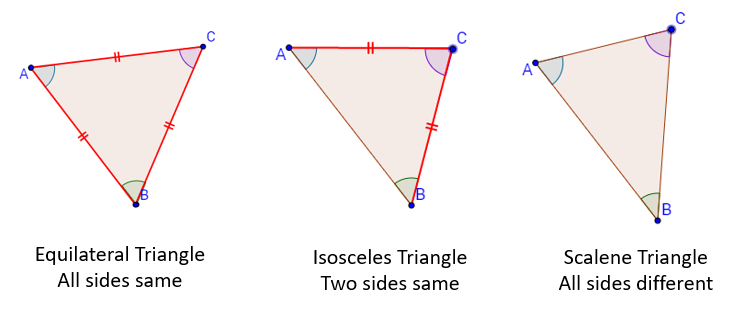### Classification of triangles based on angles

• Acute-angled: A triangle with three acute angles.
• Right-angled: A triangle with one right angle.
• Obtuse-angled: A triangle with one obtuse angle.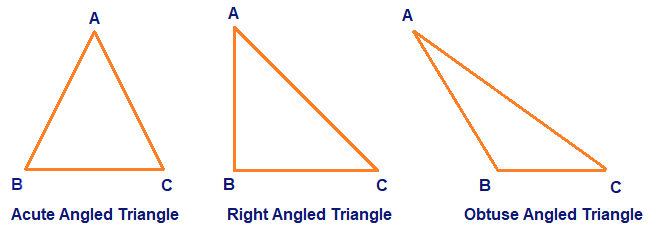#### For More Information On Classification of triangles based on angles, Watch The Below Video.## Frequently asked Questions on CBSE Class 7 Maths Notes Chapter 6: Triangles and its Properties

Q1

### What are some of the facts of triangles?

A triangle has: 1. three sides, three vertices and three angles 2. The sum of the three interior angles of a triangle is always 180° 3. The sum of the length of two sides of a triangle is always greater than the length of the third side.

Q2

### What is the ‘Angle sum property’?

The angle sum property of a triangle says that the sum of its interior angles is equal to 180°.

Q3

### What are some of the uses of triangles?

Triangles can be used to make trusses. Trusses are used in many structures, such as roofs, bridges and buildings.